# Combustion analysis

Analysis of internal combustion engines is possible with Dewesoft Combustion analysis package. During the measurement we can see typical combustion values like: Cylinder pressures, MEP values, heat release (TI, TQ, burn angles), knocking factors,….

• Required hardware - Dewesoft Sirius
• Required software - Dewesoft PROF or higher and CA option

For additional help with Combustion analysis visit Dewesoft PRO training  ->Dewesoft Web page -> PRO Training -> Combustion analysis.

To work with Dewesoft Combustion analyzer we need to do:

## Combustion analysis setup

After adding the new Combustion analysis module initial (Engine) setup screen is displayed: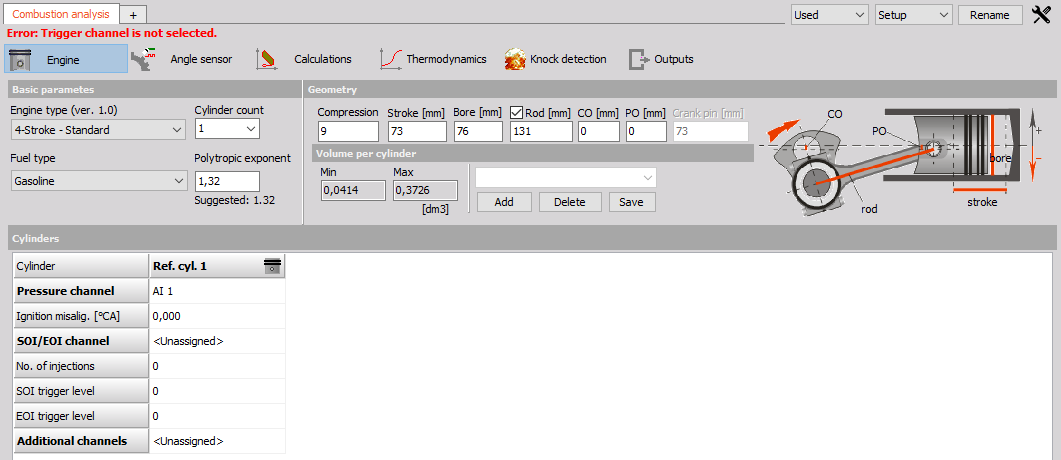We can set Combustion analysis (CA) in any way we want, but usually, the easiest way would be to select CA type and then define all settings of a particular type:

### 1. Engine setup

After selecting Engine type CA or after new CA module is added following setup screen is displayed:Settings and entered values for Engine CA type are divided into the following sections:

Basic parameters

Geometry

• Engine type
• Cylinder count
• Fuel type
• Polytropic exponent
• Compression
• Stroke
• Bore
• Rod
• CO
• PO

Volume per cylinder

• Min
• Max

Engine templates

Cylinders

• Pressure channel
• Ignition misalignment
• S01/E01 channel
• Number of injections
• S01 trigger level
• E01 trigger level

#### Basic parameters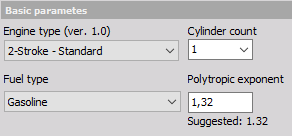Drop-down list options:

• Engine type - Engine type can be 4-stroke (for example car) or 2-stroke (motorbike).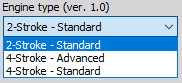• Cylinder count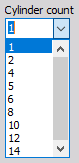• Fuel type - Fuel type defines calculation procedures, so it is important to select the correct setting.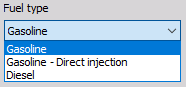Compression is the ratio between total and compressed volume:

$$R_c = \frac{V_d + V_c}{V_c}$$

$V_d$ = swept volume

$V_c$ = clearance volume

Polytropic exponent - The polytropic exponent is a fixed value for entire stroke. It is important for calculation of thermodynamic zero and for heat release. If you don’t know the polytropic exponent for your engine, take the suggested value for each engine type.

#### Geometry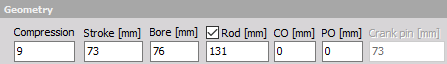On the drawing on the right, you can see what values should be entered in corresponding fields.

• Compression [bar] - Cylinder compression
• Stroke [mm] - Stroke of the piston
• Bore [mm] - Bore of the cylinder
• Rod [mm] - Rod length from the center of crankshaft to the center of piston pin
• Piston offset - PO [mm] - This is offset of the piston pin (usually zero).
• Crankshaft offset - CO [mm] - This is the offset of the crankshaft pin (usually zero).
• Crank pin [mm] - Diameter of the crankpin

#### Calculated volume

As soon as you enter the previous values and define the cylinder pressure channel, minimum and maximum volume of the engine will be calculated and shown here.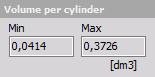#### Engine templates

All that information about engine type and geometry is stored in Dewesoft setup file. But you can save it to XML file (in Dewesoft directory CAEngines.xml) for easier handling of the geometry data.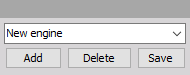Buttons:

• Delete - deletes currently selected template
• Save - saves changes to currently selected template

#### Cylinders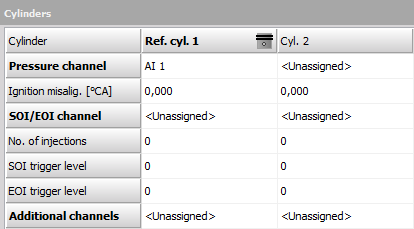• Cylinder - Reference cylinder is the one that is used for all calculations as base (it is the one which has zero misalignment). Select it by clicking in the cylinder row. In our example that is cylinder 1 (grayed).
• Pressure channel - In this row you select pressure channel for a given cylinder. At least reference cylinder needs pressure channel for correct calculations. Channels must be set correctly in the Analog setup (scaling, name, color).
• Ignition misalig. [°CA] - This is how cylinders are fired, with what delay (in degrees). You must know this for your engine.
• SOI / EOI channel - Channel for calculation of start and end of injection.
• No. of injection - Number of injections - we will have the same number of output channels for angle of start and end of injection.
• SOI trigger level - Trigger level for start of injection (always takes positive edge).
• EOI trigger level - Trigger level for end of injection (always takes negative edge).
• Additional channels - Additional channels are not used in any calculations but can be shown in displays.

Channels must be set correctly in the Analog setup (scaling, name, color).

### 2. Angle sensor setup

After selecting Angle sensor type CA following setup screen is displayed: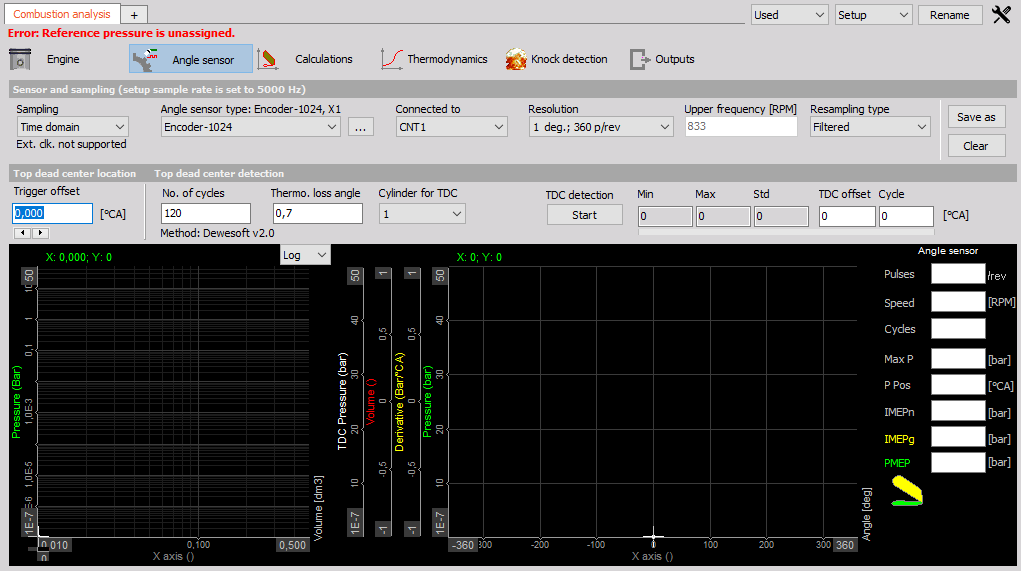Settings and entered values for this Angle sensor CA type are divided on sections:

#### Sensor and sampling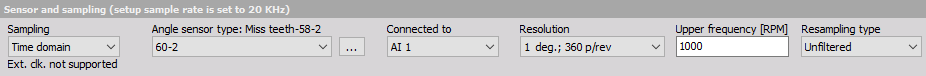Drop-down list options:

• Sampling type - Sampling type is the most important selection for the behavior of combustion analysis. Internal clock will acquire the data in the time domain and recalculate to angle domain. It will be slower, but we are able to do all time-based calculations.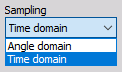• Angle sensor type - Angle sensor depends on what sensor we connected.

When three dot button is selected, the Counter sensor editor window appears to define counter sensor.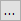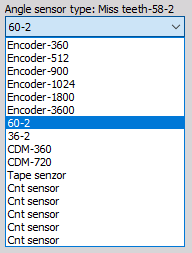• Connected to - Here we define the physical connection of the sensor. If use an external clock with CA-CPU, it will show the CA-CPU inputs. If we use the internal clock, it will offer the counter inputs for encoder and CDM sensors and analog inputs for Geartooth with double or missing teeth.• Resolution - The resolution will define the number of points per one engine revolution. A higher number will give higher accuracy, but will also bring more calculation load.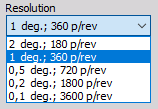For information about Angle sensor setup see  -> General mathematics modules -> Angle sensor.

Trigger offset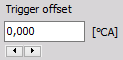Trigger offset is the offset from the trigger to top dead center of the reference cylinder.

The value in this field can be decreased and increase with buttons.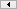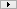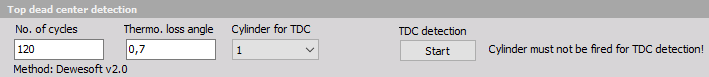• No. of cycles - Number of cycles defines the number of averages to take in TDC detection procedure.
• Thermo. loss angle - TDC detection procedure will search for pressure peak, but this peak is delayed for some angle compared to the top dead center. We enter this offset in this field.

#### TDC detection

When Start button is selected, table with values appear instead on right side table: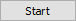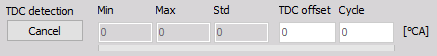Now the Start button changes to Cancel button and with selecting this we can stop TDC detection (button change again to Start).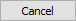When TDC detection is finished, the Average offset will be taken as the trigger offset.

### 3. Calculations setup

After selecting Calculations type CA following setup screen is displayed: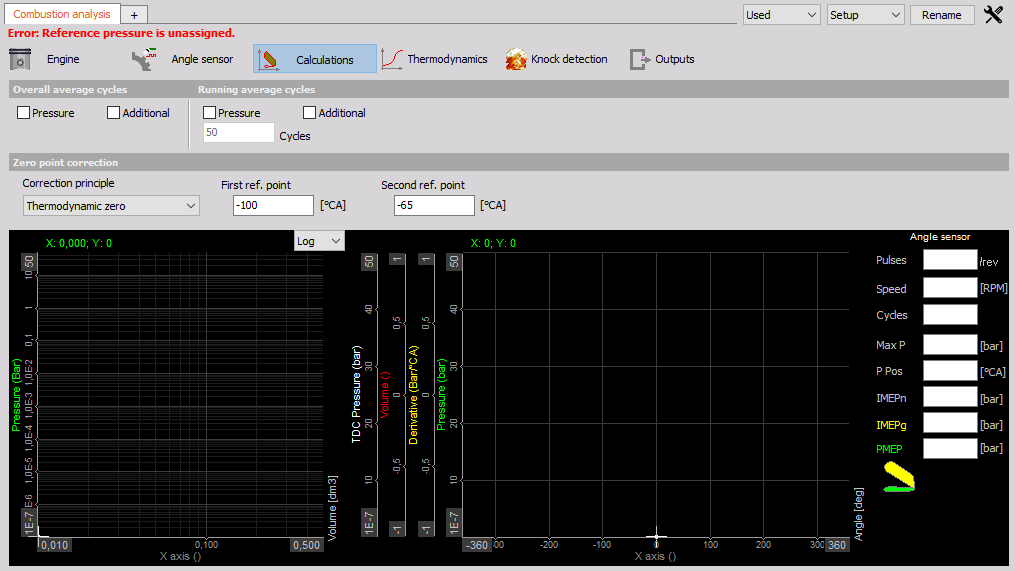Settings and entered values for this Calculations CA type are divided into sections:

#### Overall average cycles

Statistics are selected by default since these values are needed also for further calculation. The maximum pressure and position are included.

Derivation calculates the pressure derivation and adds value and position of pressure rise. When Derivation is selected, on graph appears also this value.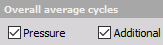#### Running average cycles

The Running average cycles calculate the mean value of the last n cycles. This basic statistic is available for the pressure and for the Additional channels of each cylinder. The result is a vector with the angle as a reference.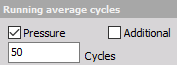#### Zero point detection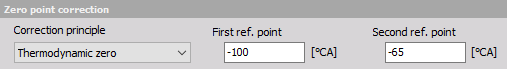Correction principle - There are three basic correction principles for the zero point correction. Thermodynamic zero assumes polytropic compression and finds the absolute pressure offset according to it. The polytropic exponent, the start and end angle for this calculation needs to fit the real compression (without ignition).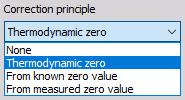### 4. Thermodynamic setup

After selecting Thermodynamics type CA following setup screen is displayed: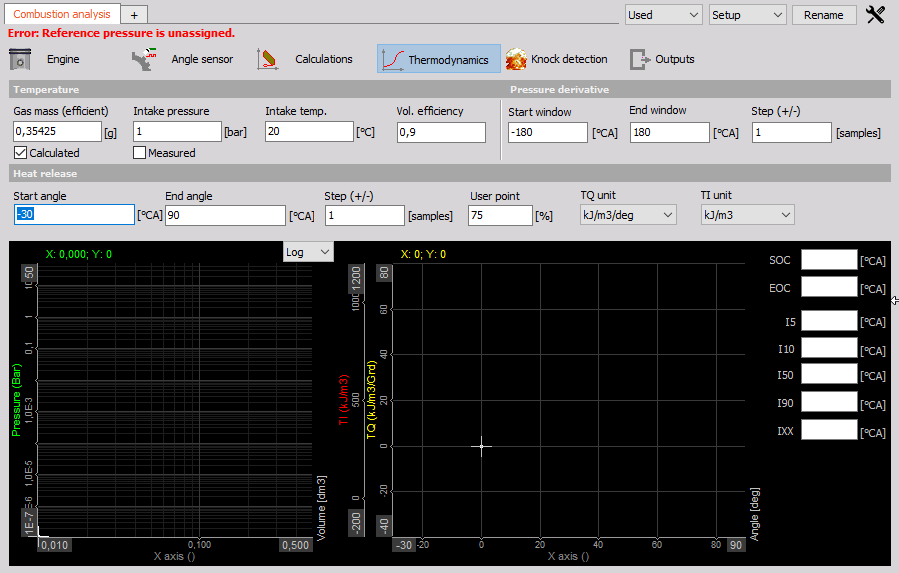Settings and entered values for this Thermodynamics CA type are divided into sections:

• Temperature
• Pressure derivative
• Gas mass (efficient)
• Intake pressure
• Intake temp
• Volumetric efficiency
• Start window
• End window
• Stef (+/-)
• Heat release
• Start angle
• End angle
• Step (+/-)
• User point
• TQ unit
• TI unit
• Display graphs
• Values table

#### Temperature

The temperature inside the combustion is calculated based on the ideal gas law and is only valid at around TDC.

Gas mass (efficient)

For the Temperature calculation, the gas mass is required. This can be either manually entered, or calculated.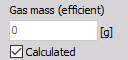Intake pressure, Intake temp and Volumetric efficiency

If from Calculated is used, the intake temperature, intake pressure, and also the volumetric efficiency (0.9= 90% filled) must be entered.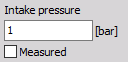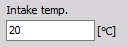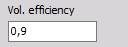#### Pressure derivative

For Pressure derivative, the start-angle, the stop-angle and also the step size must be defined.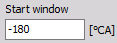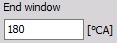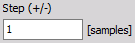#### Heat release

For setting up the Heat release calculation the start and stop angle must be defined. The typical range is from -30° to +60°. An earlier injection start angel must be set according to the real injection point.

• Start angle - The angle of the crank on the start of heat release.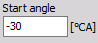• End angle - The angle of the crank on end of heat release.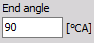• Step(+/-) - The step input field defines the calculation width: e.g. Step 1 means the calculation is based on ±1 sample (or angle resolution value). A higher value smooths the result.• User point - Heat release creates several output channels with angle values for certain amplitude values - 5, 10, 50 and 90% (called I5, I10, I50 and I90). Additionally, we can define one user point called IXX where the XX is the percentage value of the heat release defined here.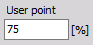• TQ unit - We can either have the physical unit for the heat release or have it expressed in percentage.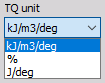• TI unit - We can either have the physical unit for the integrated heat release or have it expressed in percentage.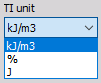### 5. Knock detection setup

After selecting Knock detection type CA following setup screen is displayed: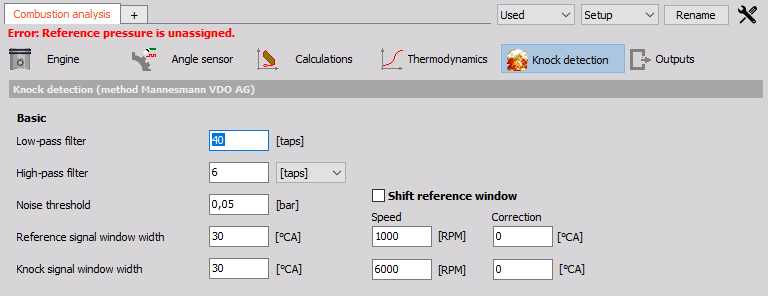Knock detection is the principle for finding the amount of knocking due to late ignition.

Settings and editable values for this Knock detection CA type are:

• Knock detection (method Mannesmann VDO AG)
• Low-pass filter
• Shift reference window
• High-pass filter
• Noise threshold
• Knock signal window width

#### Low-Pass and High-pass filter

In these fields, we define Number of taps (according to angle resolution) of the average filter to cut low and high pass part of the signal.

#### Noise threshold

Is the value which defines the lower limit for calculation of values.

#### Knock signal window width

The width of the window on the left and right side of the pressure signal.

#### Shift reference window

Is two-point linear scaling offset definition how much the calculation window is shifted for different speeds.